# 绕线巴伦器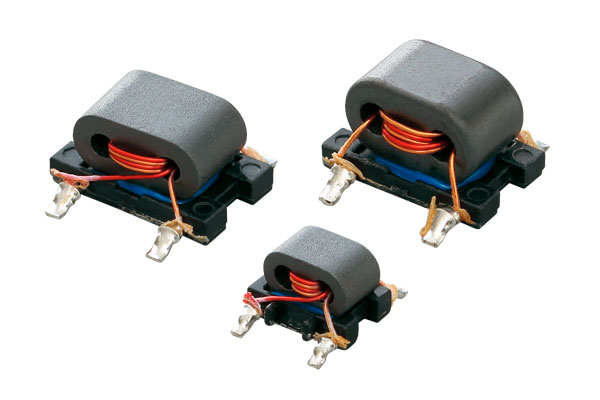##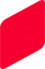规格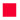DOCSIS 3.1用频率混频器用变压器巴伦变压器分配器分歧器/ 定向耦合器
• DOCSIS 3.1用
Internal connections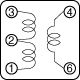Series
µiac
Winding turns
Data sheet
3T : 1Tx2 4T : 1Tx2
4T : 3Tx2
4T : 4Tx2
5T : 2Tx2
5T : 3Tx2
6T : 3Tx2
B4F
300 -
-
-
-
-
#617PT-2060=P3  -
1500 #617PT-2355=P3
-
#617PT-2288=P3
#617PT-2287=P3
#617PT-2290=P3
-
#617PT-2339=P3
B5F 800 -
#458PT-2091=P3
-
-
-
-
-

• 频率混频器用变压器
Internal connections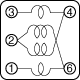Series
µiac
Winding turns
Data sheet
2 1/2Tx4 3 1/2Tx4
4 1/2Tx4
5 1/2Tx4
B4F 300 #617DB-1673=P3
#617DB-1674=P3
#617DB-1675=P3
#617DB-1714=P3
B5FL 300 #616DB-1196=P3
#616DB-1197=P3
#616DB-1198=P3
-

Internal connectionsSeries
µiac
Winding turns
Data sheet
2T:2Tx2 3T:3Tx2
4T:1Tx2
4T:4Tx2
5T:2Tx2
6T:1Tx2
6T:2Tx2 6T:3Tx2
B4F 1500 -
-
#617PT-2301=P3  -
#617PT-2304=P3  #617PT-2270=P3

#617PT-2291=P3

#617PT-2038=P3

#617PT-2323=P3

B5F 300 #458PT-1619=P3
-
-
-
#458PT-1720=P3
-
-
-
230 -
#458PT-1586=P3 -
#458PT-1587=P3
#458PT-1565=P3
-
-
＃458PT-1566=P3
1500 -
-
#458PT-2078=P3
-
-
-
#458PT-2002=P3
-
B5FL
300 #616PT-1201=P3
#616PT-1202=P3
#616PT-1203=P3
-
-
-
-

• 巴伦变压器
Internal connections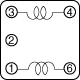Series
µiac
Winding turns
Data sheet
2 1/2Tx2 3 1/2Tx2
4 1/2Tx2
5 1/2Tx2
B4F 300 #617DB-1653=P3
#617DB-1654=P3
#617DB-1655=P3
#617DB-1724=P3
1500 #617DB-1643=P3
#617DB-1644=P3
#617DB-1645=P3
#617DB-1646=P3
B5FL 300 #616DB-1191=P3
#616DB-1192=P3
#616DB-1193=P3
#616DB-1194=P3

• 分配器
Internal connections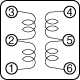Series
µiac
Winding turns
Data sheet
1-2=1T, 2-3=3T, 4-6=5-6=2T 1-2=1T, 2-3=4T, 4-6=5-6=3T
B4F
300 #617DS-1676=P3
#617DS-1711=P3
1500 #617DS-1727=P3
#617DS-1710=P3

• 分歧器/定向耦合器
Internal connections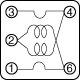Series
µiac
Winding turns
Data sheet
2 1/2T 3 1/2T
4 1/2T
5 1/2T
B4F 300 #617PS-1660=P3
#617PS-1661=P3
#617PS-1662=P3
#617PS-1663=P3
B5F 300 -
#458PS-1623=P3
#458PS-1624=P3
#458PS-1625=P3